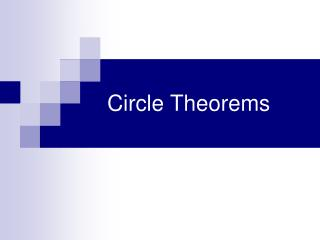DownloadDownload PresentationCircle Theorems

# Circle Theorems

Download Presentation## Circle Theorems

- - - - - - - - - - - - - - - - - - - - - - - - - - - E N D - - - - - - - - - - - - - - - - - - - - - - - - - - -
##### Presentation Transcript

1. Circle Theorems

2. Diameter Radius A Circle features……. Circumference … the distance from the centre of the circle to any point on the circumference … the distance around the Circle… … its PERIMETER … the distance across the circle, passing through the centre of the circle

3. ARC Chord Tangent A Circle features……. Major Segment Minor Segment … part of the circumference of a circle … a line joining two points on the circumference. … a line which touches the circumference at one point only From Italian tangere, to touch … chord divides circle into two segments

4. Properties of circles • When angles, triangles and quadrilaterals are constructed in a circle, the angles have certain properties • We are going to look at 4 such properties before trying out some questions together

5. An ANGLE on a chord An angle that ‘sits’ on a chord does not change as the APEX moves around the circumference Alternatively “Angles subtended by an arc in the same segment are equal” We say “Angles subtended by a chord in the same segment are equal” … as long as it stays in the same segment From now on, we will only consider the CHORD, not the ARC

6. Imagine the Chord Imagine the Chord Typical examples Find angles a and b Very often, the exam tries to confuse you by drawing in the chords YOU have to see the Angles on the same chord for yourself Angle a = 44º Angle b = 28º

7. What do you notice about the angle at the circumference? Angle at the centre A Consider the two angles which stand on this same chord It is half the angle at the centre Chord We say “If two angles stand on the same chord, then the angle at the centre is twice the angle at the circumference”

8. 272° A 136° Angle at the centre It’s still true when we move The apex, A, around the circumference As long as it stays in the same segment Of course, the reflex angle at the centre is twice the angle at circumference too!! We say “If two angles stand on the same chord, then the angle at the centre is twice the angle at the circumference”

9. a a Angle at Centre A Special Case When the angle stands on the diameter, what is the size of angle a? The diameter is a straight line so the angle at the centre is 180° Angle a = 90° We say “The angle in a semi-circle is a Right Angle”

10. A Cyclic Quadrilateral …is a Quadrilateral whose vertices lie on the circumference of a circle Opposite angles in a Cyclic Quadrilateral Add up to 180° They are supplementary We say “Opposite angles in a cyclic quadrilateral add up to 180°”

11. Questions

12. Could you define a rule for this situation?

13. Tangents • When a tangent to a circle is drawn, the angles inside & outside the circle have several properties.

14. 1. Tangent & Radius A tangent is perpendicular to the radius of a circle

15. g g 2. Two tangents from a point outside circle Tangents are equal PA = PB PO bisects angle APB 90° <APO = <BPO <PAO = <PBO = 90° 90° AO = BO (Radii) The two Triangles APO and BPO are Congruent

16. 3 Alternate Segment Theorem Alternate Segment The angle between a tangent and a chord is equal to any Angle in the alternate segment Angle in Alternate Segment Angle between tangent & chord We say “The angle between a tangent and a chord is equal to any Angle in the alternate (opposite) segment”